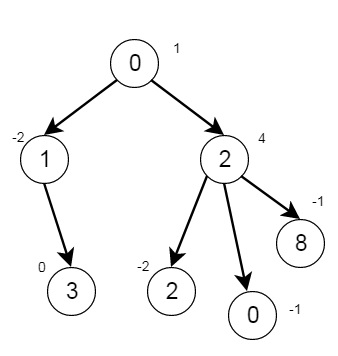# Delete Tree Nodes in C++

Suppose we have a tree, this tree is rooted at node 0, this is given as follows −

1. Number of nodes is nodes
2. Value of ith node is value[i]
3. Parent of ith node is parent[i]

We have to remove every subtree whose sum of values of nodes is 0, after doing that return the number of nodes remaining in the tree. So if the tree is like −There are 7 nodes, the output will be 2

To solve this, we will follow these steps −

• Create a map called children
• define a method called dfs(), this will take node, an array value and graph
• temp := a pair (value[node], 1)
• for i in range 0 to size of graph[node]
• temp2 := dfs(graph[node, i], value, graph)
• increase first by first of temp2, increase second by second of temp2
• if first of temp is 0, then ans := ans – second of temp, set second of temp := 0
• return temp
• From the main method, it will take nodes, parents array and values array
• n := number of values present in values array
• ans := n
• define an array graph of size n + 1
• for i in range 1 to n – 1
• insert i into graph[parent[i]]
• dfs(0, value, graph)
• return ans

## Example(C++)

Let us see the following implementation to get a better understanding −

Live Demo

#include <bits/stdc++.h>
using namespace std;
class Solution {
public:
map <int, int> children;
int ans;
pair <int, int> dfs(int node, vector<int>& value, vector <int> graph[]){
pair <int, int> temp = {value[node], 1};
for(int i = 0; i < graph[node].size(); i++){
pair <int, int> temp2 = dfs(graph[node][i], value, graph);
temp.first += temp2.first;
temp.second += temp2.second;
}
if(temp.first == 0){
ans -= temp.second;
temp.second = 0;
}
return temp;
}
int deleteTreeNodes(int nodes, vector<int>& parent, vector<int>& value) {
int n = value.size();
ans = n;
children.clear();
vector < int > graph[n + 1];
for(int i = 1; i < n; i++){
graph[parent[i]].push_back(i);
}
dfs(0, value, graph);
return ans;
}
};
main(){
vector<int> v1 = {-1,0,0,1,2,2,2};
vector<int> v2 = {1,-2,4,0,-2,-1,-1};
Solution ob;
cout << (ob.deleteTreeNodes(7,v1, v2));
}

## Input

7
[-1,0,0,1,2,2,2]
[1,-2,4,0,-2,-1,-1]

## Output

2